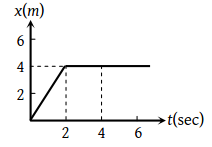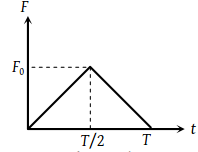A man fires a bullet of mass 200 g at a speed of 5 m/s. The gun is of one kg mass. By what velocity the gun rebounds backwards ?

(1) 0.1 m/s

(2) 10 m/s

(3) 1 m/s

(4) 0.01 m/s

Concept Questions :-

Linear momentum
High Yielding Test Series + Question Bank - NEET 2020

Difficulty Level:

A body of mass M at rest explodes into three pieces, two of which of mass M/4 each are thrown off in perpendicular directions with velocities of 3 m/s and 4 m/s respectively. The third piece will be thrown off with a velocity of

(1) 1.5 m/s

(2) 2.0 m/s

(3) 2.5 m/s

(4) 3.0 m/s

Concept Questions :-

Linear momentum
High Yielding Test Series + Question Bank - NEET 2020

Difficulty Level:

In the figure given below, the position-time graph of a particle of mass 0.1 Kg is shown. The impulse at t = 2 sec is(1) 0.2 kg m sec–1

(2) –0.2 kg m sec–1

(3) 0.1 kg m sec–1

(4) –0.4 kg m sec–1

Concept Questions :-

Linear momentum
High Yielding Test Series + Question Bank - NEET 2020

Difficulty Level:

A particle of mass m moving with velocity u makes an elastic one dimensional collision with a stationary particle of mass m. They are in contact for a very short time T. Their force of interaction increases from zero to F0 linearly in time T/2, and decreases linearly to zero in further time T/2. The magnitude of F0 is(1) mu/T

(2) 4mu/T

(3) mu/2T

(4) None of these

Concept Questions :-

Linear momentum
High Yielding Test Series + Question Bank - NEET 2020

Difficulty Level:

A force of 50 dynes is acted on a body of mass 5 g which acts for an interval of 3 seconds, then impulse is

(1) 0.15 × 10–3 N-s

(2) 0.98 × 10–3 N-s

(3) 1.5 × 10–3 N-s

(4) 2.5 × 10–3 N-s

Concept Questions :-

Linear momentum
High Yielding Test Series + Question Bank - NEET 2020

Difficulty Level:

In an explosion a body breaks up into two pieces of unequal masses. In this

(1) Both parts will have numerically equal momentum

(2) Lighter part will have more momentum

(3) Heavier part will have more momentum

(4) Both parts will have equal kinetic energy

Concept Questions :-

Linear momentum
High Yielding Test Series + Question Bank - NEET 2020

Difficulty Level:

Two bodies of masses m1 and m2 have equal kinetic energies. If p1 and p2 are their respective momentum, then ratio p1 : p2 is equal to

(1) ${m}_{1}:{m}_{2}$

(2) ${m}_{2}:{m}_{1}$

(3) $\sqrt{{m}_{1}}:\sqrt{{m}_{2}}$

(4) ${m}_{1}^{2}:{m}_{2}^{2}$

Concept Questions :-

Linear momentum
High Yielding Test Series + Question Bank - NEET 2020

Difficulty Level:

A light and a heavy body have equal momenta. Which one has greater K.E

(1) The light body

(2) The heavy body

(3) The K.E. are equal

(4) Data is incomplete

Concept Questions :-

Linear momentum
High Yielding Test Series + Question Bank - NEET 2020

Difficulty Level:

If the momentum of a body is increased n times, its kinetic energy increases-

(1) n times

(2) 2n times

(3) $\sqrt{n}$ times

(4) n2 times

Concept Questions :-

Linear momentum
High Yielding Test Series + Question Bank - NEET 2020

Difficulty Level:

A bomb of 12 kg explodes into two pieces of masses 4 kg and 8 kg. The velocity of 8kg mass is 6 m/sec. The kinetic energy of the other mass is

(1) 48 J

(2) 32 J

(3) 24 J

(4) 288 J

Concept Questions :-

Linear momentum